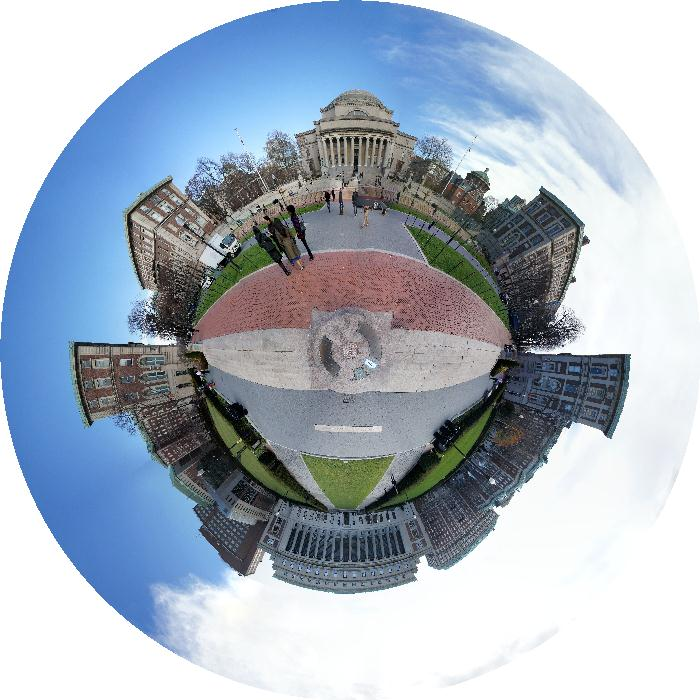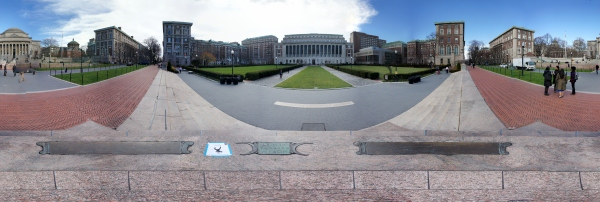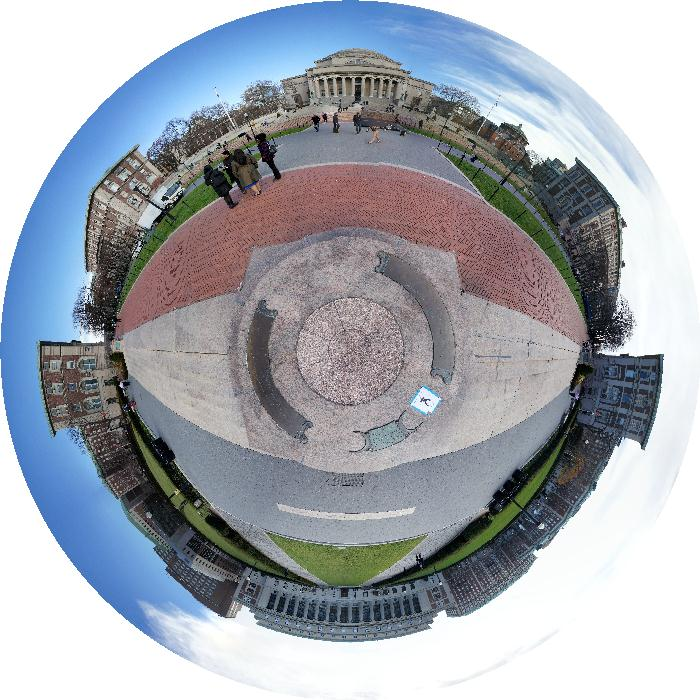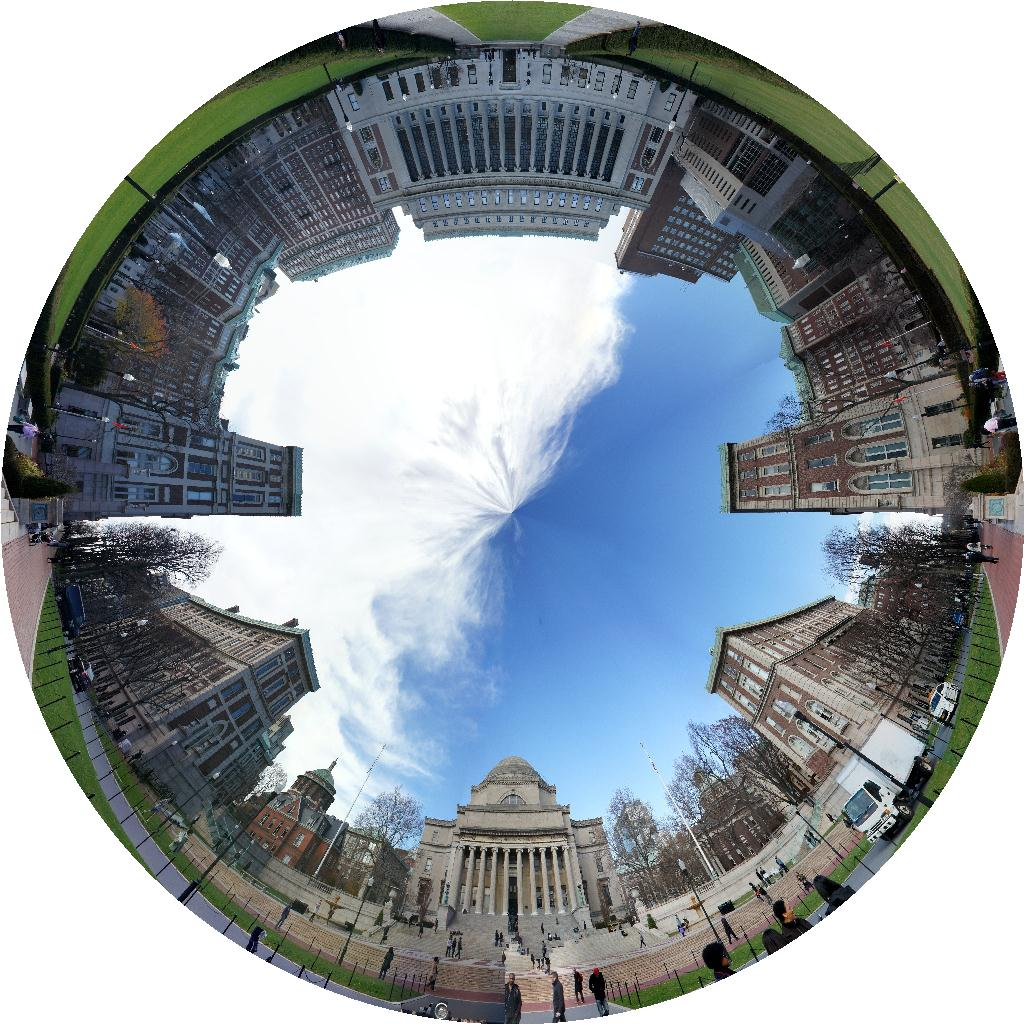## Build a Small-World Fish-Eye Style Panorama

Sometimes a small-world panorama is quite stunning. For comparison, a regular panorama is shown below.Spent about half an hour to do experiments and finally get a program to convert to regular panorama to small-world style.

The key is coordinate system transform, from rectangular to polar. This is pretty intuitive to derive. For a point $(\psi, r)$ in the target image, its correspondence in the original panorama is $(\psi w / (2\pi), h - ry / r_0)$, where h, w is the height and width of the original image, and $r_0$ is the expected radius of the output.Note we may need a nonlinear mapping for the radius to make the panorama more balanced. Here I choose the function $r_0^{-\epsilon} r^\epsilon$, where $\epsilon$ is a parameter to be tuned, controlling the "height" of the camera from the ground. You can see the difference between the two images above.

Another style of small-world panorama is also easy to derive and implement as the following photo.